This web page is part of a three-part tutorial on radar absorbing materials used for radar cross-section reduction.

Part one is on fundamentals of electromagnetic waves.

Part two is on radar cross-section physics You are here!

Part three is on radar absorbers and absorption mechanisms.

Basic RCS reduction approaches

Metals and dielectrics

Reflection coefficient

### Introduction

Before we can discuss radar cross-section reduction, we first must examine the physics behind radar cross-section.

In directional antenna systems, most of the radiated power is sent into a forward cone: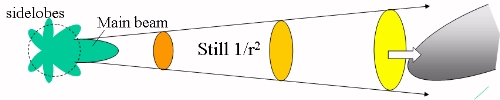The power density (S) reaching a target is equal to :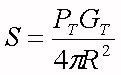Where GT is the gain of the antenna and PT is the power radiated by the antenna.

The incident wave excites currents on the scatterer (the target you are trying to illuminate) which then becomes an antenna re-radiating with its own antenna pattern. The power returned (PR) is measured by the radar cross-section (RCS), denoted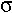. We defineas the area of an ideal "mirror" that reflects that amount of power back to the source.

##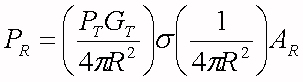Here AR is the receiving area of the antenna. The echo goes as 1/R4. To drop the detection range by a factor of two, the required return loss off of the object can be calculated: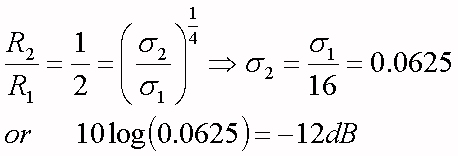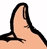Microwaves101 Rule of Thumb

An absorber with a 12 dB return loss allows the scatterer to get twice as close to the radar before being detected, compared to an object with 0 dB return loss.

Radar cross-section is dominated by shape, because it's the shape that governs how much of the incident power is intercepted and sent back, as illustrated in the three examples below, where the objects are assumed to be large compared to the incident wavelength. The example on the left illustrates mirror-like reflection or specular reflection, here the reflection is proportional to 1/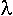2. The middle example shows the amplitude of the reflections from a vertical cylinder. Looking straight onto the cylinder produces maximum backscatter. However, at certain angles other than 90 degrees there can be also considerable reflection as shown. For a cylinder, the reflected signal is proportional to 1/. On the right is a sphere, which also reflects back a signal proportional to the radius of the sphere (and not a function of wavelength).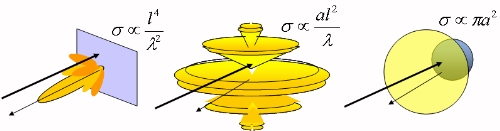Orientation of the shape is critical. The worst case when the wave is incident perpendicular to a flat part of the surface, which results in specular reflection. Canting the surfaces redirects this echo.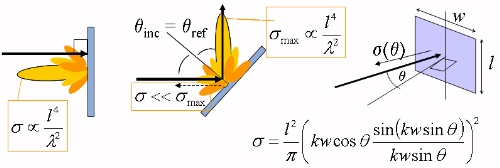Beware of "corner reflectors" when you are designing for low radar cross-section, as shown below. Inside corners can increase your radar cross-section much more than you'd imagine.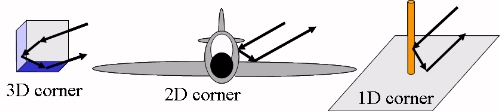If the specular echo is redirected, the remainder is the next greatest contributor to radar cross-section. It is due to diffraction, which is caused by discontinuities of the surface. Discontinuities imply a change in the boundary conditions; and boundary conditions are what govern the distribution of the fields in the first place. Metals at radio frequency behave nearly like perfect electric conductors (PECs). This invokes a well-known boundary condition of Maxwell's equations: the tangential E-field must go to zero on the surface of a conductor.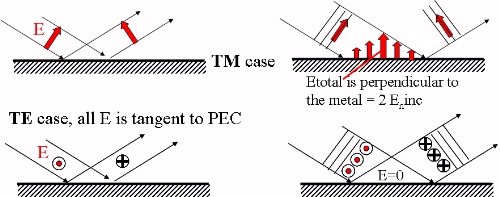Where did the energy go if total ETE =>0? It goes into the H-field: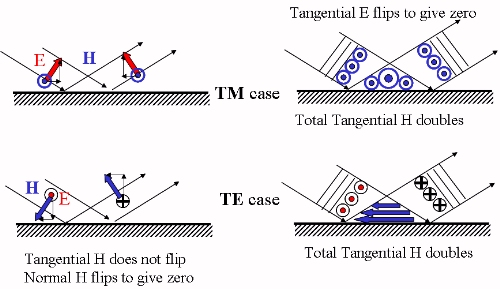On metal surfaces, E total is always perpendicular to the surface, and H total is parallel to the surface. On the shadow side the E-field attaches and travels along the surface at the speed of light. Equal and opposite charges created at the leading edge create almost no scatter. On the illuminated side we get a running (traveling) wave consisting of incident and reflected waves until it runs out of surface. Then it scatters strongly.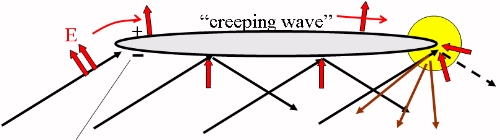V-Pol, TM incidence on wing

For horizontal polarization (H-pol) it is the leading edge of an object such as of an aircraft wing that scatters strongly. The leading edge gets a very strong current induced in it whose job is to create the wave that exactly cancels the E field tangential to the metal. Clearly the radar cross-section reduction job is different for leading edges and trailing edges. Because Etotal = 0, there is no significant diffraction off the trailing edge.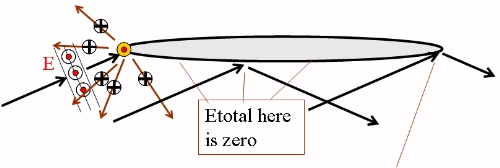The color figures below show two-dimensional finite-difference time domain simulations. The white rectangle surrounding the target is a teleportation boundary separating the total field region from the scattered field region outside. This is a standard way of examining scattering phenomena because we are interested in the field scattered by the object out to the far field. Inside the total field region, in the illuminated side, you will see standing waves, the interference between the incident and scattered field. Inside the total field region then you will also see the "apparent wavelengths". If the wave is running at an angle to the surface, the apparent wavelength is longer (the wave is fast) compared to free space (similar to the guide wavelength in waveguide). But in the shadow side, the only waves are creeping waves and diffracted waves whose wavelength is that of free space.

The figures show the field strength of the field perpendicular (out of) the figure. For TE it is E field strength for TM it is H field strength. The colors indicate + and - so you can visualize the wavelength (one full red and one full blue region). The color has been saturated to highlight the phasefronts rather than given a continuous spectrum of color from red to blue.

Scattered field view, 45o incidence onto a perfect electrical conductor, TM incidence: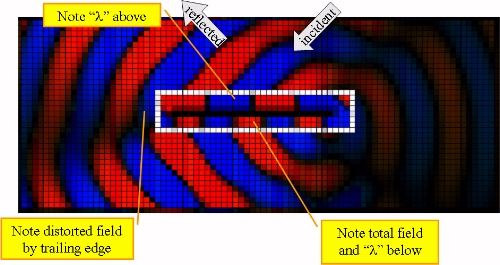Scattered field view, 45o incidence onto a perfect electrical conductor, TE incidence: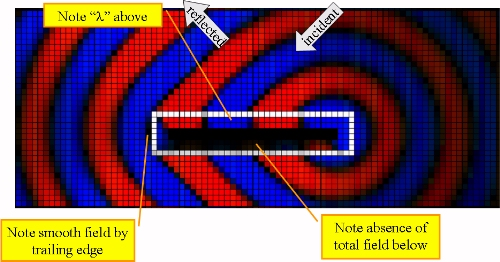Total field view, 45o TM incidence onto a hole in a PEC: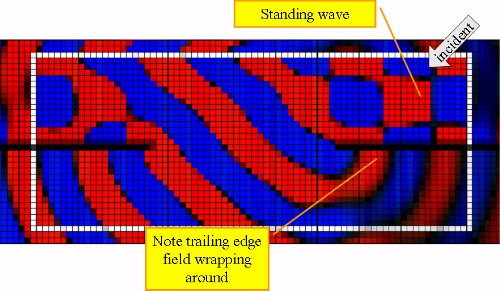Total field view, 45o TE incidence onto a hole in PEC: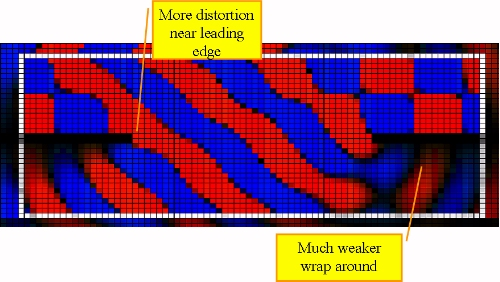Diffraction is a challenge because it can go in many directions. The only general rule that edge diffraction obeys is that angle of incidence = angle of re-radiation cone. Shaping helps to some extent but the forward scattered rays can be reflected downstream and come back as backscatter. Therefore the discontinuity must be softened or the surface covered with a surface wave absorber.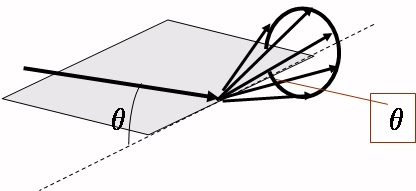### Basic RCS reduction approaches

Softening with resistive cards (R-cards) is one method of RCS reduction. A tapered-conductivity R-card breaks up scattering into many small echoes instead of a large single one.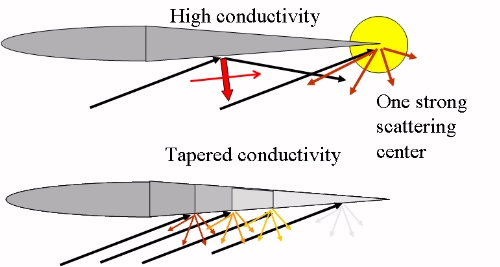The best surface-wave absorber is magram (magnetic radar absorbing material). There are two fields available to defeat at the surface of a PEC: the normal E-field, and the tangential H-field. Magram attacks the strong tangential H-field. Magram works very fast in a few inches, but magram is very heavy, it contains iron particles embedded in synthetic rubber. The actual weight depends on the application and is frequency-specific: magram at cell-phone frequencies can be 0.3 inches (1 centimeter) thick! Higher frequencies permit thinner magram.

A surface of resistive hairs would have loss in the right direction to take advantage of the vertical E-field, but too little current flows along the hairs because the hairs end in a open circuit. Thus such an absorber requires a longer distance to attenuate a creeping waveâ€¦ several feet, and is not practical.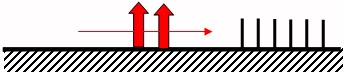As seen in the upper figure below, the trailing edge echo always contains the reverberations (multiple reflections of surface traveling waves) of the plate. These reverberations create interference as they radiate from both ends of the strip. The r-card kills the surface wave, so there is no more distortion of the field after it is generated the first time at the edge itself. Therefore, we see the smooth, cylindrical backscatter in the second figure below.

For TE there are no TE surface waves on metal so we see no reverberations. The leading edge echo is clearly damped immediately by the graded resistive edge.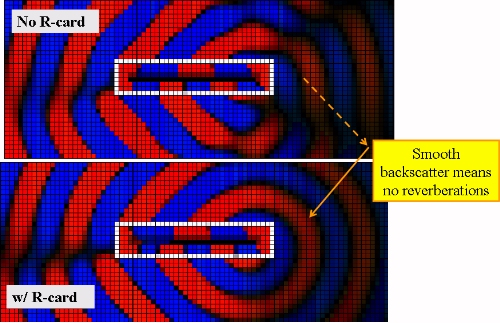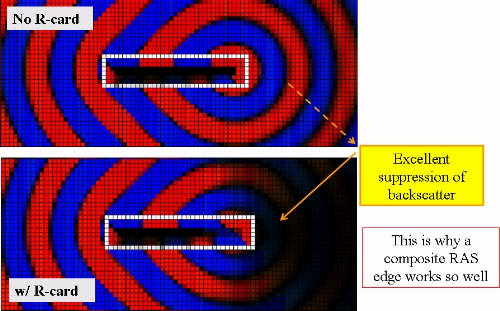Ideal magram covering the whole object for TM incidence: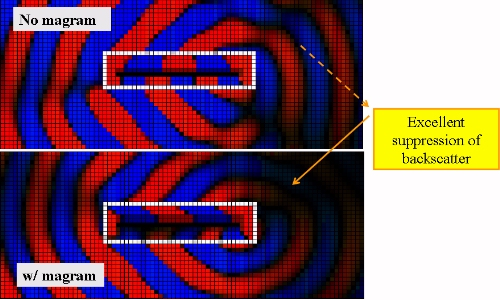### Metals and dielectrics

The behavior of a material is governed by the impedance of the material:

Impedance Z or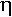=377 ohms x (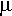R/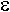R)1/2

A perfect electrical conductor (metal) has,â€ ~ infinity (=â€™-jâ€, whereâ€=/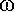0, andis conductivity mhos/m). Therefore a PEC is a zero impedance object.

What about nonmetal objects? IfR>1 andR ~ 1, a dielectric is a low impedance object: Z<377 ohms. Dielectrics tend to behave like PECs, because their impedance can be quite low depending on the dielectric constant.

IfR>R , Z>377 ohms, and you are considering a magneto-dielectric, high impedance object. You can likewise define magnetic conductivity as given by m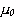â€=m/0, wherem is magnetic conductivity (ohms/m) and a PMC has,â€~ =infinity.

### Reflection coefficient

For PECs or PMCs ,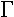(the reflection coefficient) = 100% or 0 dB. At normal incidence on a half-space of the material,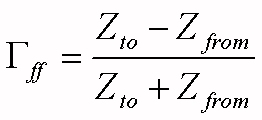Note that< 0 for dielectrics, and> 0 for magnetics. The sign tells you whether the tangential E field flips in phase or not . For Teflon (R = 2), gamma~ -15 dB. For FR4 (R = 4.8),~ = -8.5 dB

Things get even more interesting off normal.

Fresnelâ€™s Laws point out the difference between TE and TM incidence on a dielectric interface. The reason for the dependence on angle of incidence is that the boundary conditions of Maxwellâ€™s equations apply to the tangential fields. Relative to the material surface, a TM wave has part of its E-field tangential and all its H-field tangential. A TE wave has part of its H-field tangential and all its E-field tangential. Therefore the impedance that matters is the so-called transverse impedance.

Define a coordinate system and plane of incidence: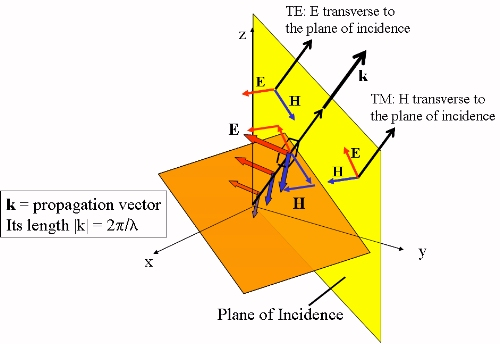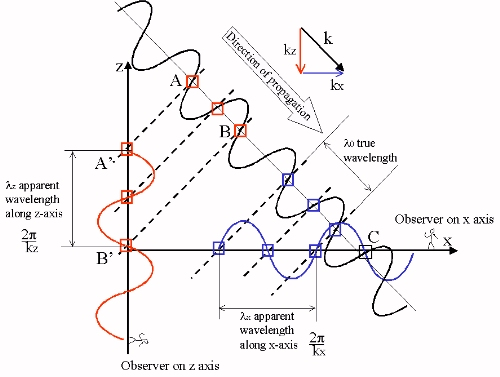The transverse or tangential impedance is the ratio of the tangential fields.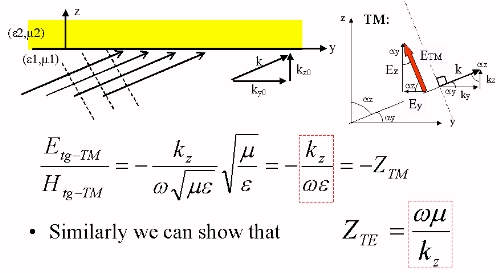Then the reflection coefficient is defined the same way as before. But because kz changes with angle of incidence the two impedances (to and from) also change, but at different rates.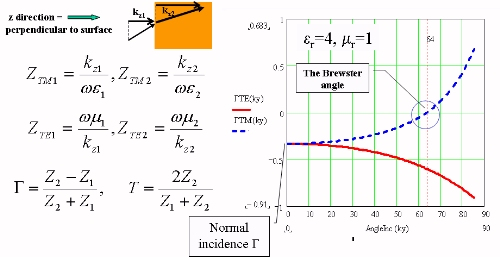This behavior is often plotted as || versus angle. This emphasizes that for dielectrics the TM front face reflectivity drops with angle except near grazing.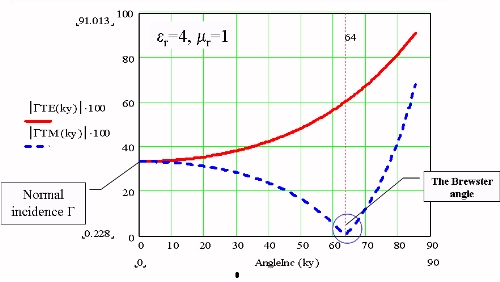Fresnelâ€™s Laws mean radomes depolarize antenna radiation. They also mean that when a material or structure (RAS) is specified for off-normal performance, TM is always easier because the wave gets into the absorbing material easier, to be converted from RF energy into heat.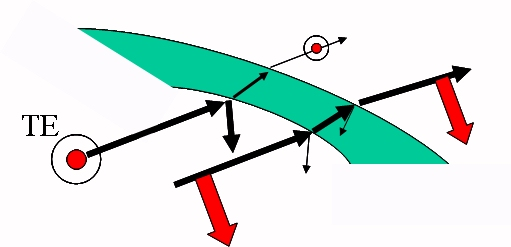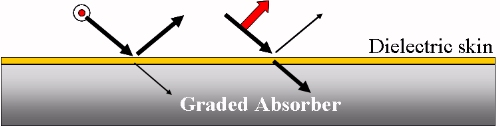In addition to reflecting and refracting a wave, a dielectric can trap a wave. These are called surface waves and they travel at a speed between the speed of light in air and the speed of light in the dielectric, carrying a significant amount of energy in the air outside. A creeping wave on metal always sheds energy away and gets weaker. A surface guided wave on a dielectric skin can travel a long distance losing almost no energy and then hit a discontinuity and re-radiate. If there is underlying metal (or graphite epoxy) then only the TM wave is bound. It must be attenuated.

For instance, put a dielectric coating on the bottom side of the PEC plate. The echo is brighter because the creeping wave doesn't just leave the plate, it reflects and bounces around.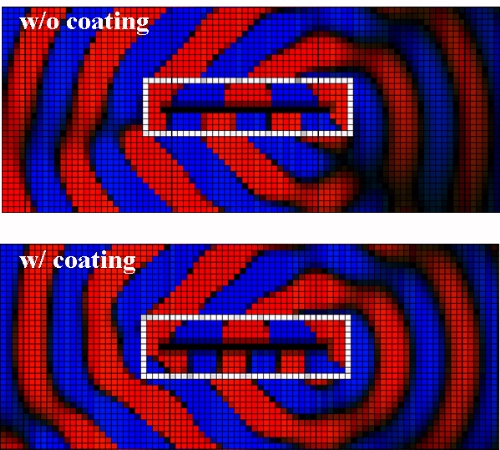Time to summarize some radar cross-section phenomena: specular reflection means 100% reflection off metal, <100% off dielectric. Specular reflection can be redirected by shaping, avoiding corner reflectors, and the use of broadband absorbers. Diffraction off discontinuities can be redirect by shaping, shed off by bending or employing magram or tapered R-cards. Traveling waves include direct illumination running wave, creeping wave on metal, trapped guided wave on dielectric. Magram can counter traveling waves, or you can consider making the guiding dielectric lossy.

There is one more thing to consider: resonant scatterers are a combination of discontinuities that allow the echo to buildup. Natural resonators include antennas and cavities. In the figures below a wave of wavelength = 10 cells in FDTD is incident at 45 degrees onto a metal groundplane with two notches (perhaps seams at a door). The apparent wavelength (y)along the surface is then about 14 cells (1.414*10) . Let's examine the scattered field. When the distance between the notches is comparable to half of the apparent wavelength along the surface we get strong scattering, as shown in the third picture (separation= 8 cells).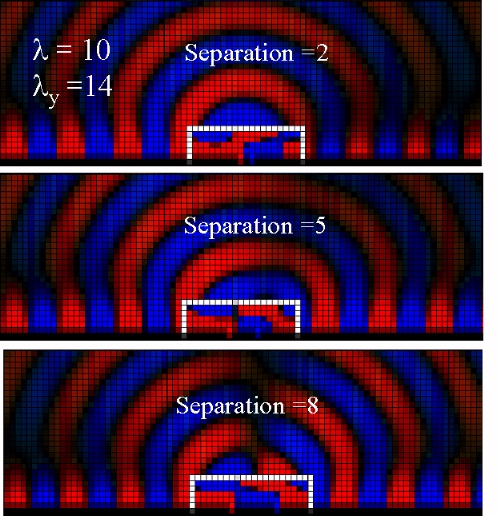To damp the resonance you can cover the notches with magram. You can see in the figure below that the backscatter is reduced.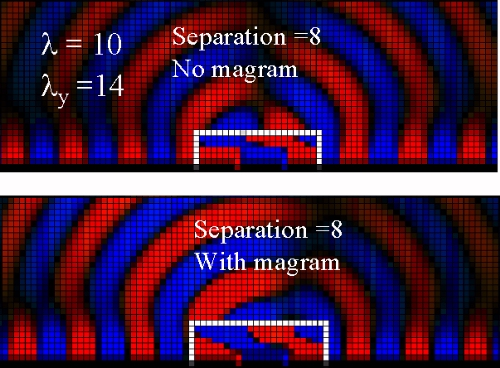Much of the technical material on this page was prepared by Dr. Rudy Diaz of Arizona State University, for ARC Technologies, Inc.

Author : Dr. Rudy Diaz